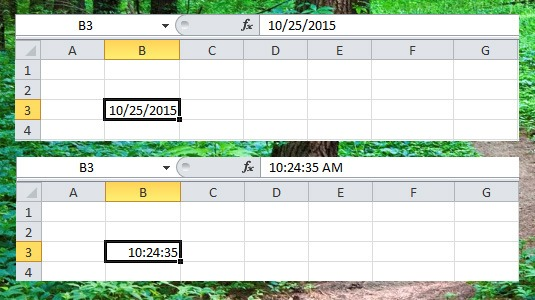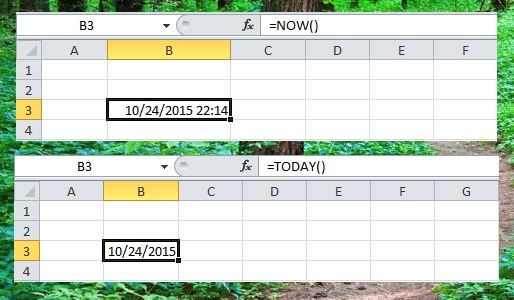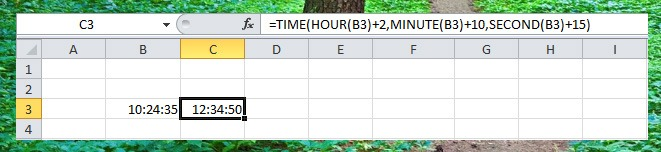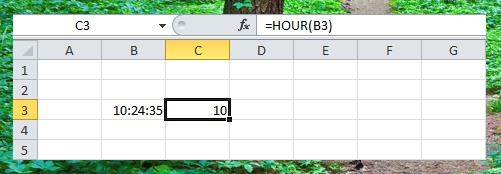# Date and Time Functions

Date and time are very important in Excel project, like in making a timesheet, you need to get the elapsed time to compute the total number of regular hours and overtime.

In this lesson you will learn some basic date and time functions like, date, month, day and time. Let’s see how to use these functions.

To enter Date in excel use the “/” or “-” to separate the month, date and year (e.g. 10/25/2015 or 10-25-2015) now depending on your cell date format, it may appear differently when you press enter. To add Time on excel, use “:” to separate the hours, minutes and seconds, like in the example below.Date Functions

Use the Date functions to add or subtract a number of years, months or days, In the example below, we will add 3 years, subtract 3 months and add 4 days of the given date on cell B3.If you only want to add or subtract days, you can use this simple formula.Current Date and Time

In a spreadsheet, you can easily add the current date and time using a NOW() functions and the current date without time using TODAY() function.Time Functions

To add numbers or hours, minutes or seconds you can use the TIME() functions. In the example below, we will add 2 hours, 10 minutes and 15 seconds on the given time in cell B3.Hour Functions

To return the hour at the given time, you can use HOUR() functions.